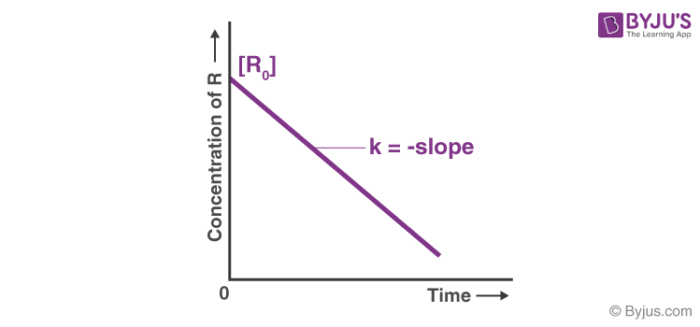# Zero Order Reaction

## What is a Zero Order Reaction?

Zero-order reaction is a chemical reaction wherein the rate does not vary with the increase or decrease in the concentration of the reactants. Therefore, the rate of these reactions is always equal to the rate constant of the specific reactions (since the rate of these reactions is proportional to the zeroth power of reactants concentration).

### Differential and Integral Form of Zero Order Reaction

The Differential form of a zero order reaction can be written as:

Rate =

$$\begin{array}{l}\frac{-dA}{dt} = k[A]_{0} = k\end{array}$$

Where ‘Rate’ refers to the rate of the reaction and ‘k’ is the rate constant of the reaction.

This differential form can be rearranged and integrated on both sides to get the required Integral form as shown below.

Rate =

$$\begin{array}{l}\frac{-d[A]_{0}}{dt}= k\end{array}$$

Multiplying both sides with ‘-dt’, we get:

$$\begin{array}{l}d[A]= -kdt\end{array}$$

Integrating on both sides, we get:

$$\begin{array}{l}\int_{[A]_{0}}^{[A]}d[A] = -\int_{0}^{t}kdt\end{array}$$

Where [A]0 is the initial concentration of the reactant [A] at time t=0. Solving for [A], we get:

$$\begin{array}{l}[A] = [A]_{0} – kt\end{array}$$

Which is the required integral form. This form enables us to calculate the population of the reactant at any given time post the start of the reaction.

### Graph of Zero Order Reaction

The integral form of zero order reactions can be rewritten as

$$\begin{array}{l}[A] = – kt + [A]_{0}\end{array}$$

Comparing this equation with that of a straight line (y = mx + c), an [A] against t graph can be plotted to get a straight line with slope equal to ‘-k’ and intercept equal to [A]0 as shown below.## Half-Life of a Zero Order Reaction

The timescale in which there is a 50% reduction in the initial population is referred to as half-life. Half-life is denoted by the symbol ‘t1/2’.

From the integral form, we have the following equation

$$\begin{array}{l}[A] = [A]_{0} – kt\end{array}$$

Replacing t with half-life t1/2 we get:

$$\begin{array}{l}\frac{1}{2}[A] = [A]_{0} – kt_{1/2}\end{array}$$

Therefore, t1/2 can be written as:

$$\begin{array}{l}kt_{1/2} = \frac{1}{2}[A]_{0}\end{array}$$

And,

$$\begin{array}{l}t_{1/2} = \frac{1}{2k}[A]_{0}\end{array}$$

It can be noted from the equation given above that the half-life is dependent on the rate constant as well as the reactant’s initial concentration.

## Recommended Videos## Examples of Zero Order Reaction

The following reactions are examples of zero order reactions that are not dependent on the concentration of the reactants.

• The reaction of hydrogen with chlorine (Photochemical reaction).

$$\begin{array}{l}H_{2}(g) + Cl_{2} (g)\overset{hv}{\rightarrow} 2HCl (g)\end{array}$$

• Decomposition of nitrous oxide over a hot platinum surface.

$$\begin{array}{l}2N_{2}O \overset{Pt(hot)}{\rightarrow} 2N_{2} + O_{2}\end{array}$$

• Iodization of Acetone (In H+ ion rich medium)

$$\begin{array}{l}CH_{3}COCH_{3} + I_{2} \overset{H^{+}}{\rightarrow} ICH_{2}COCH_{3} + HI\end{array}$$

Reactions wherein a catalyst is required (and is saturated by reactants) are generally zero order reactions. The unit of the rate constant in a zero order reaction is given by concentration/time or M/s  where ‘M’ is the molarity and ‘s’ refers to one second.

## Frequently Asked Questions In Exam:

### What is meant by the Zero Order Reaction?

Zero order reactions are those in which the concentration of the reactants does not change over time and the concentration rates remain constant.
A → Product

### What are the units of k for a Zero Order Reaction?

The rate constant of the reaction is denoted by k.
In a zero-order reaction, the rate constant is expressed as concentration/time or M/s, where ‘M’ is the molarity and ‘s’ is one second.
∴ k = mol L–1 s–1 is the unit of rate constant.

### What is the rate law for a Zero Order Reaction?

For a zero-order reaction, the rate law is rate = k, where k is the rate constant. In the case of a zero-order reaction, the rate constant k will be expressed in concentration/time units, such as M/s.

### How do you know if it’s a Zero Order Reaction?

If the reactant concentration increases, the reaction has zero-order kinetics. It has first-order kinetics if it has no effect. The reaction has second-order kinetics if increasing the reactant decreases the half life.

### Are zero order reactions fast?

A zero-order reaction, unlike the other orders of reaction, has a rate that is independent of the reactant concentration (s). As a result, increasing or decreasing the concentration of the reacting species has no effect on the reaction rate.

## Practise Questions of Zero Order Reaction

1. What is zero order kinetics?
2. What are the units of a Zero Order Reaction?
3. What is the zero-order rate law?
4. How do you know if a graph is a zero or second-order?
5. What is the equation for the half-life of a zero-order process?
6. What is the zero-order integrated rate law?